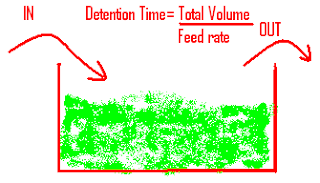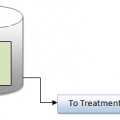## How to Calculate Detention Time of Any Tank | Basin

In waste water treatment plant you are hearing frequently the word of Detention Time.OK before to calculate the Detention Time of any tank or basin,First we should understood about that one and how it is important one.
Detention time is the length of time water is retained in a vessel or basin or the period from the time the water enters a settling basin until it flows out the other end. When calculating unit process detention times, we are calculating the length of time it takes the water to flow through that unit process.
Detention times are normally calculated for the following basins or tanks:• Flash mix chambers (seconds)
• Flocculation basins (minutes)
• Sedimentation tanks or clarifiers (hours)
• Wastewater ponds (days)
• Oxidation ditches (hours)

Now we will see with simple example
To calculate the detention period of a tank, the volume of the tank, must first be obtained. Using a tank 70 ft long (L), 25 ft wide (W), and 12 ft deep (D), the volume (V ) would be:
V = L x W x D
V = 70 ft x 25 ft x 12 ft
V = 21,000 ft3
Gallons = V x 7.48 [1ft3=7.48gallons]
So Total Volume of the tank in Gallons = 21,000 x 7.48 = 157,080 gallons

If we assume that the plant filters 300 gpm, then we have 157,080 ÷ 300 = 524 minutes, or roughly 9 hours, of detention time. Stated another way, the detention time is the length of time theoretically required for the coagulated water to flow through the tank / basin.

Key Point: If detention time is desired in minutes, then the flow rate used in the calculation should have the same time frame (cfm or gpm, depending on whether tank volume is expressed as cubic feet or gallons). If detention time is desired in hours, then the flow rate used in the calculation should be cfh or gph.

Detention time (hrs)=volume of tank (gal) or (litre) / flow rate (gph)or (lph)## Author:T.P.Sivanandan

#### Why Fine Bubbles are Better than Coarse bubble in Aeration Tank#### How to Calculate Lime Dosage Requirement in Sedimentation Process## 1 thought on “How to Calculate Detention Time of Any Tank | Basin”

1.fitrasyofyan says:

very good and clearly